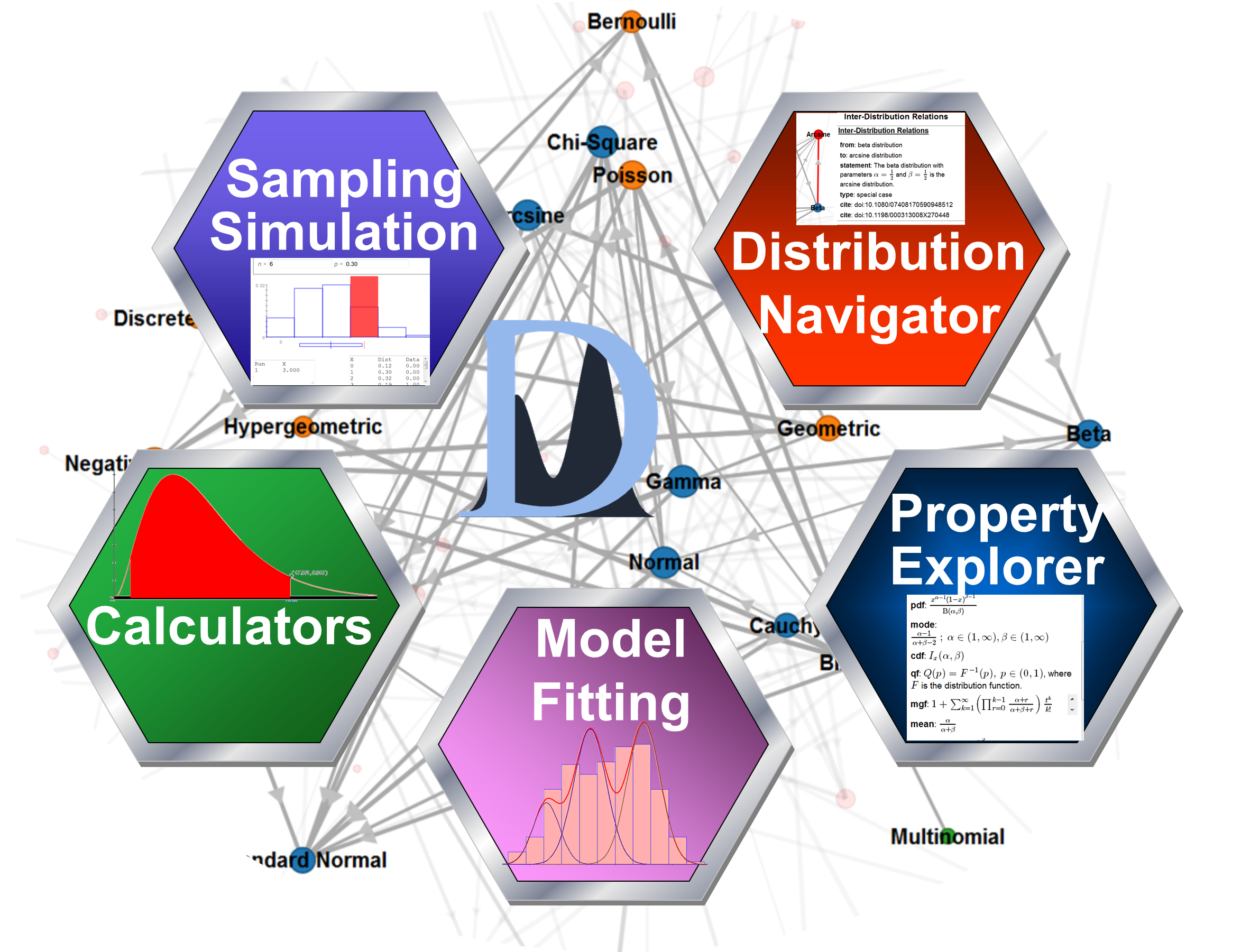The Probability Distributome Project is an open-source, open content-development project for exploring, discovering, navigating, learning, and computational utilization of diverse probability distributions. A probability distribution is a function that assigns probabilities to events. For example, flipping a fair coin generates 2 possible equally likely outcomes (head and tail), and rolling a single loaded 6-face die generates 6 possible outcomes, each having a chance to be observed proportionally-equal to the die’s loading factors. Usually, a probability distribution function is defined in the context of a random variable, or a vector, and provides likelihoods that the random variable may be observed within a specific sub-set of the space of its possibilities. For instance, throwing a dart at a dartboard generates a score (integer value), indicating the landing location’s proximity to the center of the dartboard (bulls eye), which naturally leads to a definition of the probability distribution of the dart landing in a specific area. Probability distributions are mathematically rich objects that give rise to other mathematical functors. Depending on the nature of the space where a probability distribution is defined (discrete, continuous, univariate, multivariate, Euclidian and non-Euclidean, etc.), it naturally leads to various functors like  probability mass or density function, distribution function, quantile function, probability and moment generating functions, and so on. In its most general form, a probability distribution is defined as mapping between a measurable space and the unitary interval.The interactive Distributome graphical user Navigator (requires JavaScript-enabled browser) facilitates the traversal, discovery and exploration of the Distributome meta-data, as well as enables the community of users to review, update, expand and submit meta-data revisions to improve this infrastructure. Examples of core Distributome functionality includes:
• Visually traversal of the space of all well-defined (named) distributions;
• Exploration of the interrelationships between different distributions;
• Distribution search by keyword, property and type;
• Retrieval of qualitative (e.g., analytic form of density function) and quantitative (e.g., critical and probability values) information about each distribution;
• Discovery of references and additional distribution resources.
• Interactive utilization of various probability distribution calculators, simulators and experiments.